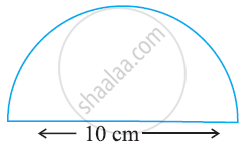# Find the Perimeter of the Adjoining Figure, Which is a Semicircle Including Its Diameter. - Mathematics

Find the perimeter of the adjoining figure, which is a semicircle including its diameter.#### Solution

Length of curved part = πr

= 22/7 xx 5

= 15.71 cm

Total perimeter = Length of curved part + Length of diameter

= 15.71 + 10 = 25.71 cm

Concept: Area of Circle
Is there an error in this question or solution?

#### APPEARS IN

NCERT Class 7 Maths
Chapter 11 Perimeter and Area
Exercise 11.3 | Q 7 | Page 223